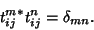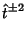## Double Contraction Relation

A Tensoris said to satisfy the double contraction relation whenThis equation is satisfied bywhere the hat denotes zero trace, symmetric unit Tensors. These Tensors are used to define the Spherical Harmonic Tensor.

Arfken, G. Alternating Series.'' Mathematical Methods for Physicists, 3rd ed. Orlando, FL: Academic Press, p. 140, 1985.